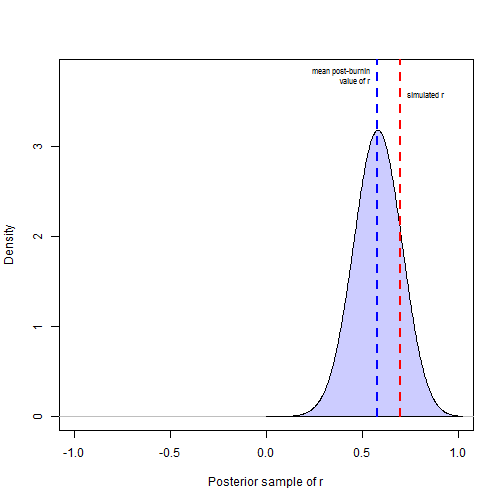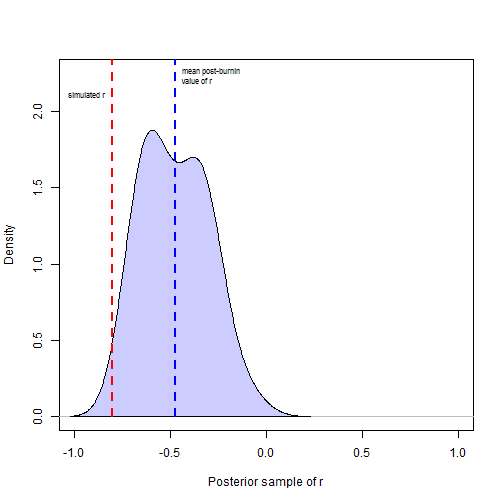## Thursday, November 9, 2017

### Update & new S3 methods for `threshBayes`

The phytools function `threshBayes` implements a Bayesian MCMC version of Joe Felsenstein's 2005 & 2012 model for analyzing the correlation between a discrete & a continuous character, or between two discrete characters, under the threshold model from evolutionary quantitative genetics.

Mine is a relatively simple function. Whereas Joe's implementation, in the PHYLIP program THRESHML uses an EM algorithm and can accept an arbitrary number of characters of each type, phytools `threshBayes` can only take one binary character and one continuous character or two binary characters (or two continuous characters, although this doesn't make much sense).

The threshold model is a model in which the state of a discrete character is determined by an underlying, unobserved trait called liability. When the liability crosses a particular value (the threshold) the discrete character changes state. This makes defining the correlation between two discrete characters very easy - it is merely the correlation between the underlying liabilities. Similarly, the correlation between a discrete & a continuous trait can be defined merely as the correlation between the liabilities of the former & the observed values of the latter.

`threshBayes` is an old function of the phytools package, but today I updated it to have a little more flexibility in the input data format (for instance, it before could only take a numeric matrix with the discrete character coded as 0s and 1s - now it can take arbitrarily coded discrete characters as factors in a data frame). I also added nice S3 `print` and `plot` methods. In this case, the former just prints a small summary of the MCMC sample (handy in Bayesian MCMC methods in which the function output can often be a very large object), and the latter plots a posterior density of the measured correlation (r) between characters. This is usually the thing we will be most interested in for this method.

Here is a demo using two simulated datasets - one consisting of a discrete character that was simulated to be positively correlated with a second, continuous character. The second demo consists of two discrete traits whose liabilities were simulated with a positive correlation, but then I flipped the order of one of the two characters - which should thus result in a measured negative evolutionary correlation between the two characters.

``````library(phytools)
## first our data
tree
``````
``````##
## Phylogenetic tree with 200 tips and 199 internal nodes.
##
## Tip labels:
##  t34, t41, t99, t100, t97, t98, ...
##
## Rooted; includes branch lengths.
``````
``````head(X)
``````
``````##              V1 V2
## t34  2.44237717  B
## t41  2.24849079  B
## t99  3.55026650  B
## t100 3.79849104  B
## t97  1.33254157  B
## t98  0.08405969  B
``````
``````head(Y)
``````
``````##      V1 V2
## t34   a  d
## t41   a  d
## t99   b  c
## t100  b  c
## t97   a  d
## t98   a  d
``````
``````## now run the MCMC for data matrix X
mcmc.X<-threshBayes(tree,X,control=list(sample=200,quiet=TRUE),
ngen=200000)
``````
``````## Starting MCMC....
## Done MCMC.
``````
``````mcmc.X
``````
``````##
## Object of class "threshBayes" consisting of a matrix (L) of
## sampled liabilities for the tips of the tree & a second matrix
## (par) with the sample model parameters & correlation.
##
## Mean correlation (r) from the posterior sample is: 0.54705.
##
## Ordination of discrete traits:
##
##  Trait 2: A <-> B
``````
``````plot(mcmc.X,bw=0.1)
lines(rep(0.7,2),c(0,par()\$usr),lwd=2,lty="dashed",col="red")
text(x=0.7,y=0.9*par()\$usr,"simulated r",pos=4,cex=0.7)
````````````## the MCMC for Y
mcmc.Y<-threshBayes(tree,Y,control=list(sample=200,quiet=TRUE),
ngen=200000)
``````
``````## Starting MCMC....
## Done MCMC.
``````
``````mcmc.Y
``````
``````##
## Object of class "threshBayes" consisting of a matrix (L) of
## sampled liabilities for the tips of the tree & a second matrix
## (par) with the sample model parameters & correlation.
##
## Mean correlation (r) from the posterior sample is: -0.46684.
##
## Ordination of discrete traits:
##
##  Trait 1: a <-> b
##  Trait 2: c <-> d
``````
``````plot(mcmc.Y,bw=0.1)
lines(rep(-0.8,2),c(0,par()\$usr),lwd=2,lty="dashed",col="red")
text(x=-0.8,y=0.9*par()\$usr,"simulated r",pos=2,cex=0.7)
``````The data for this example were simulated as follows:

``````library(phytools)
tree<-pbtree(n=200)
X<-as.data.frame(sim.corrs(tree,matrix(c(1,0.7,0.7,1),2,2)))
X[]<-as.factor(threshState(X[],setNames(c(0,Inf),c("A","B"))))
Y<-as.data.frame(sim.corrs(tree,matrix(c(1,0.8,0.8,1),2,2)))
Y[]<-as.factor(threshState(Y[],setNames(c(0,Inf),c("a","b"))))
Y[]<-as.factor(threshState(Y[],setNames(c(0,Inf),c("d","c"))))
``````